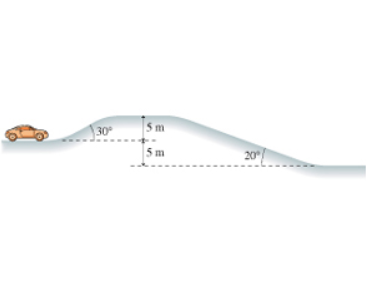# Problem: A 1400 kg car is approaching the hill shown in the figure at 13.0m/s when it suddenly runs out of gas. (Figure 1)What is the car's speed after coasting down the other side? in m/s

###### FREE Expert Solution

Kinetic energy:

$\overline{){\mathbf{K}}{\mathbf{E}}{\mathbf{=}}\frac{\mathbf{1}}{\mathbf{2}}{\mathbf{m}}{{\mathbf{v}}}^{{\mathbf{2}}}}$

Potential energy:

$\overline{){\mathbf{U}}{\mathbf{=}}{\mathbf{m}}{\mathbf{g}}{\mathbf{h}}}$

Conservation of energy:

KE0 + U0 = KEf + Uf

Uf = 0 (because hf = 0)

• m = 1400 kg
• v0 = 13.0m/s
• h0 = 5 m
• g = 9.8 m/s2

(1/2)mvf2 = (1/2)mv02 + mgh###### Problem Details

A 1400 kg car is approaching the hill shown in the figure at 13.0m/s when it suddenly runs out of gas. (Figure 1)

What is the car's speed after coasting down the other side? in m/s